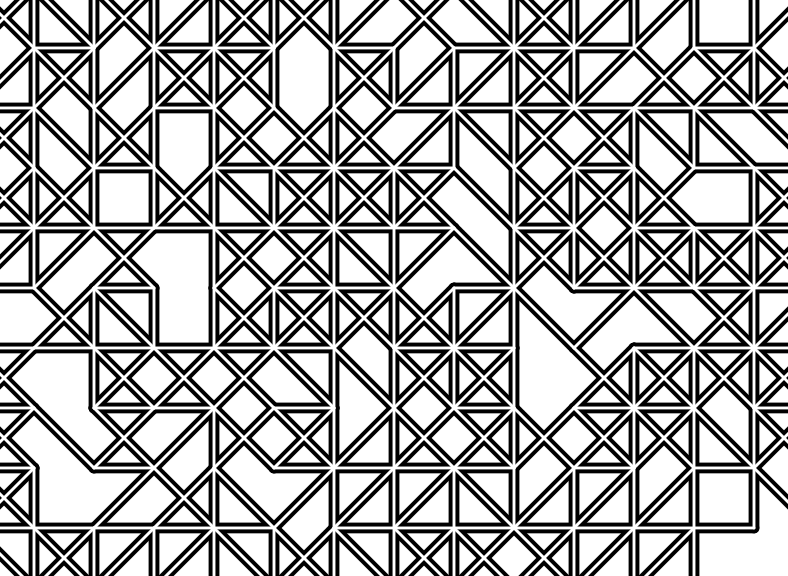# mathr / blog / #

## ReCode Project - Traveling Through the Square SeriesThe ReCode Project is a community-driven effort to preserve computer art by translating it into a modern programming language. -- ReCode Project

An admirable aim, but unfortunately they chose Processing, when other more elegant languages are available. I had a browse through the index of artworks, and Roger Coqart's Traveling Through the Square Series caught my eye. I wasn't particularly taken by Sermad Buni's implementation on the site, the output seemed buggy and the code complicated and long. So I decided to write my own using the Haskell programming language and the wonderful Diagrams library.

I'm using the painter's algorithm to draw hollow edges, which is much easier than trying to draw the separate shapes that appear. I draw all the edges with a thick black line in one go, then overlay with thin white lines in the same locations. To determine the edges, the image is divided into a grid of cells, each having four possible edges: at the top and left and both diagonals. The boundaries of the image are treated specially to have a solid edge, the inner edges have a fixed probability of being present or absent.

import Diagrams.Prelude
import Diagrams.Backend.SVG.CmdLine (defaultMain)

import Data.Array (array, indices, range, (!))
import Data.List.Split (chunksOf)
import System.Random (newStdGen, randoms)

main = newStdGen >>= defaultMain . diagram

diagram g =
let a = grid g
in  (flatten thin white a <> flatten thick black a) # bg white

grid g =
let bs = ((1, 1), size)
in  array bs \$ range bs zip chunksOf 4 (coins probability g)

flatten w c a
= mconcat (map (cell a) (indices a))
# lw w # lc c # lineCap LineCapRound

cell a ix@(i, j) =
let [top, left, down, up] = a ! ix
top'   = j == 1 || top
left'  = i == 1 || left
right  = i == fst size
bottom = j == snd size
in  mconcat
[ top'   ? edge (i    , j    ) (i + 1, j    )
, left'  ? edge (i    , j    ) (i    , j + 1)
, down   ? edge (i    , j    ) (i + 1, j + 1)
, up     ? edge (i    , j + 1) (i + 1, j    )
, right  ? edge (i + 1, j    ) (i + 1, j + 1)
, bottom ? edge (i    , j + 1) (i + 1, j + 1)
]

a ? b = if a then b else mempty

edge (a, b) (c, d) =
let p (x, y) = p2 (fromIntegral x, fromIntegral y)
in  p (a, b) ~~ p (c, d)

coins p g = map (< p) (randoms g)

size :: (Int, Int)
size = (32, 18)

probability :: Double
probability = 0.7

You can download this Haskell source code, and some earlier versions are on lpaste if you're into code evolution.